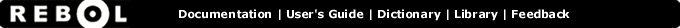# // - Function Summary

## Summary:

Returns the remainder of first value divided by second.

// value1 value2

## Arguments:

value1 - The value1 argument. (must be: number pair char money time tuple)

value2 - The value2 argument. (must be: number pair char money time tuple)

## Description:

Returns the value of the remainder after the first number is divided by the second. If the second number is zero, an error will occur.

 ``` print 123 // 10 3```

 ``` print 25:32 // 24:00 1:32```

If the first value is positive, then the returned remainder is nonnegative.

If the first value is negative, then the returned remainder is nonpositive.

## Related:

* - Returns the first value multiplied by the second.
/ - Returns the first value divided by the second.
remainder - Returns the remainder of first value divided by second.